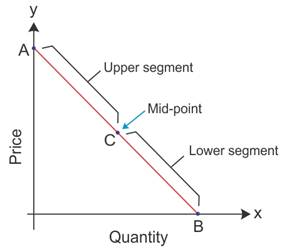SSC BOARD PAPERS IMPORTANT TOPICS COVERED FOR BOARD EXAM 2024

### The Geometric method measures the elasticity of demand

The Geometric method measures the elasticity of demand at different points on the demand curve and is also known as the Point method of measuring the elasticity of demand.

Let us consider the figure given below, where AB is a demand curve. C is the specific point on the demand curve. It divides the demand curve into two segments, upper segment CA and lower segment CB. Elasticity of demand at point C is the ratio between lower segment and upper segment.

ed= CB (lower segment from C) / CA (Upper segment from C)The elasticity of demand at different points on a straight line demand curve can be derived by this method.
1. Elasticity is zero at point B of the demand curve, where the demand curve touches the x-axis.
2. ed= 0/BA
3. ed= 0
4. Elasticity is infinity at point A of the demand curve, where the demand curve touches the y-axis.
5. ed= BA/0
6. ed= 0
7. Elasticity is unity at the mid-point C of the demand curve, where the lower segment is equal to the upper segment of the demand curve.
8. ed= CB/CA
9. ed= 1
10. Elasticity is greater than unity at any point (L) to the left of the mid-point of the demand curve.
11. ed= LB/LA
12. ed> 1
13. Elasticity is less than unity at any point (M) to the right of the mid-point of the demand curve.
14. ed= MB/MA
15. ed< 1